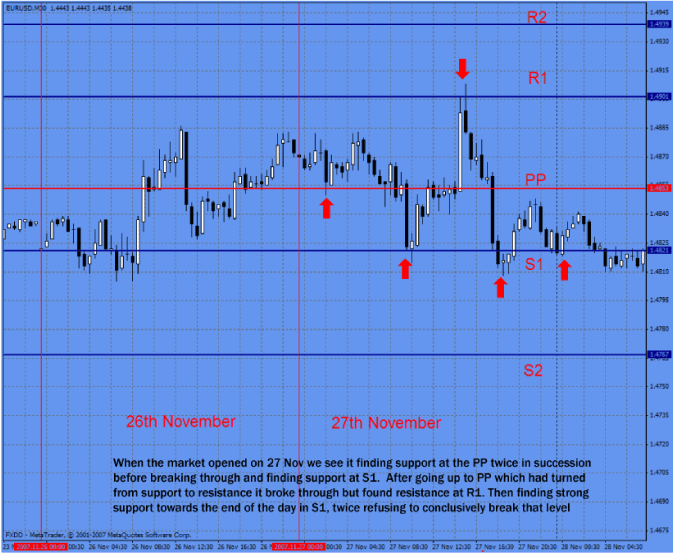# Mapping our Time Frame-Section 2: Pivot Points

Section 2: Pivot Points

In a few words, a pivot point (PP) is a level in which the sentiment of traders and investors changes from bull to bear or vice versa.

Why PP works?

They work simply because many traders and investors (including bank and institutional traders) use and trust them. It is known by every trader that the pivot point is an important measure of strength or weakness of any market.

There are several ways to calculate the pivot point. The method we found to have the most accurate results is calculated by taking the average of the high, low and close of a previous period (or session).

Warning – some pretty boring maths ahead. The good news is that almost all charting platforms will automatically calculate this for you and draw the lines on in whatever pretty colour you like. But as this is the ADVANCED course we think you should have a basic idea of how they are calculated.

Pivot point (PP) = (High + Low + Close) / 3

Take for instance the following EUR/USD information from the previous session:

Open: 1.2386

High: 1.2474

Low: 1.2376

Close: 1.2458

The PP would be,

PP = (1.2474 + 1.2376 + 1.2458) / 3 = 1.2436

So, what does this number tell us? It simply tells us that if the market is trading above 1.2439, Bulls are winning the battle pushing the prices higher. In addition, if the market is trading below this 1.2439 the bears are winning the battle pulling prices lower. In both cases this condition is likely to sustain until the next session.

Since the Forex market is a 24hr market (no close or open from day to day) there has been an ongoing battle deciding at what times we should take the open, close, high and low from each session. From our point of view, the times that produce more accurate predictions is taking the open at 00:00 GMT and the close at 23:59 GMT (obviously the high and low in between those hours).

Besides the calculation of the PP, there are other support and resistance levels that are calculated using the PP as a reference.

Resistance 2 (R2 ) = PP + (R1 – S1)

Resistance 1 (R1) = (PP * 2) - L

Pivot Point (PP) = (H + L + C)/3

Support 1 (S1) = (PP * 2) – H

Support 2 (S2) = PP – (R1 – S1)

Where, H is the High of the previous period

L is the low of the previous period

C is the close price

Continuing with the example above, PP = 1.2436

R2 = 1.2436 + (1.2496 – 1.2398) = 1.2534

R1 = (1.2436 * 2) – 1.2376 = 1.2496

PP = 1.2436

S1 = (1.2436 * 2) - 1.2474 = 1.2398

S2 = 1.2436 – (1.2496 – 1.2398) = 1.2338

These levels are supposed to mark support and resistance levels for the current session.

In the next chart we have calculated the PP and the support and resistance levels for November 27th.

S2 = 1.4767

S1 = 1.4821

PP = 1.4854

R1 = 1.4901[Chart 1]

Vertical lines separate sessions (26th and 27th of November). As we can see, the market went rapidly below the PP level and stopped right at S1, then the broke again the PP and it was stopped again by R1. Once again, it broke the PP and stalled around S1.

On the example above, the PP was calculated using information of the previous session (previous day). This way we could see possible intraday resistance and support levels. But it can also be calculated using the previous weekly or monthly data to determine such levels. By doing so, we are able to see the sentiment over longer periods of time. In addition, we can see possible levels that might offer support and resistance throughout the week or month. Calculating the weekly or monthly pivot point is mostly used by long term traders, and as we said, it gives us a good idea about the longer term trend.

Got it? Good - there will be questions on this in the quiz later…

As mentioned; all these mapping indicators are available on a point and click basis on almost all charting packages as they are so commonly used (therefore have such intrinsic power). We prefer and choose to use MetaTrader 4 and will go into more detail about that package later.